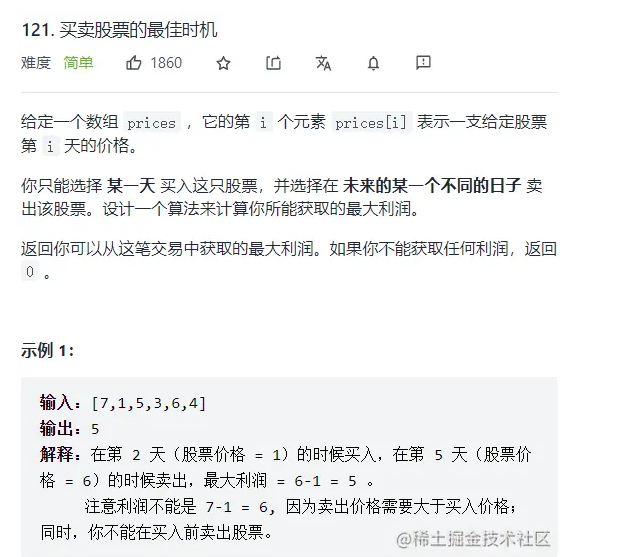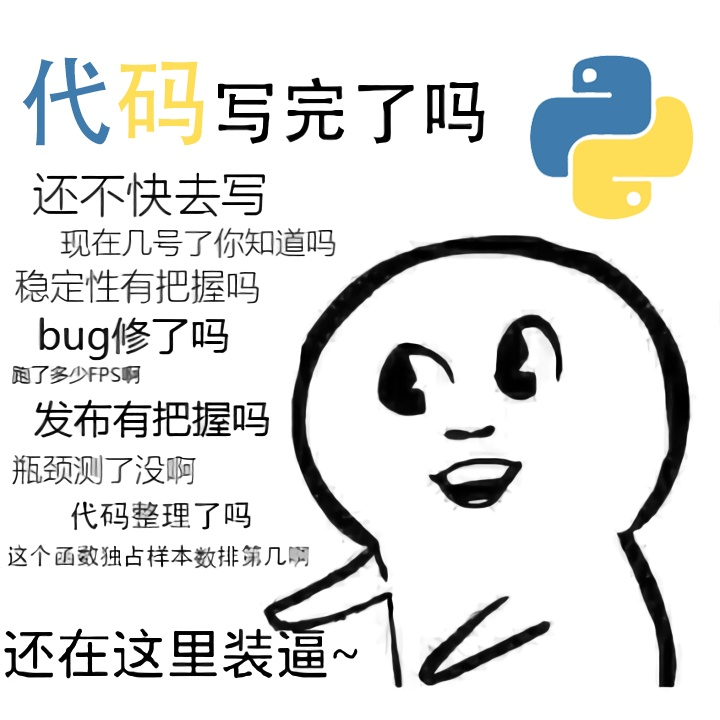+关注继续查看

# 1.题目

You are a professional robber planning to rob houses along a street. Each house has a certain amount of money stashed, the only constraint stopping you from robbing each of them is that adjacent houses have security system connected and it will automatically contact the police if two adjacent houses were broken into on the same night.

Given a list of non-negative integers representing the amount of money of each house, determine the maximum amount of money you can rob tonight without alerting the police.

# 2.代码

public class Solution {
public int rob(int[] nums) {
int[][] num=new int[nums.length+1];

for(int i=1;i<=nums.length;i++){
num[i]=Math.max(num[i-1],num[i-1]);
num[i]=nums[i-1]+num[i-1];
}
return Math.max(num[nums.length],num[nums.length]);
}
}

# 1.题目

A message containing letters from A-Z is being encoded to numbers using the following mapping:

'A' -> 1
'B' -> 2
...
'Z' -> 26


Given an encoded message containing digits, determine the total number of ways to decode it.

For example,
Given encoded message "12", it could be decoded as "AB" (1 2) or "L" (12).

The number of ways decoding "12" is 2.

# 2.代码

public class Solution {
public int numDecodings(String s) {
int n = s.length();
if (n == 0) return 0;

int[] memo = new int[n+1];
memo[n]  = 1;
memo[n-1] = s.charAt(n-1) != '0' ? 1 : 0;

for (int i = n - 2; i >= 0; i--)
if (s.charAt(i) == '0') continue;
else memo[i] = (Integer.parseInt(s.substring(i,i+2))<=26) ? memo[i+1]+memo[i+2] : memo[i+1];

return memo;
}
}

# 1.题目

Given a string s and a dictionary of words dict, determine if s can be segmented into a space-separated sequence of one or more dictionary words.

For example, given
s = "leetcode",
dict = ["leet", "code"].

Return true because "leetcode" can be segmented as "leet code".

# 2.代码

public class Solution {
public boolean wordBreak(String s, Set<String> dict) {

boolean[] f = new boolean[s.length() + 1];
Arrays.fill(f, false);
f = true;
for(int i=1; i <= s.length(); i++){
for(int j=0; j < i; j++){
if(f[j] && dict.contains(s.substring(j, i))){
f[i] = true;
break;
}
}
}

return f[s.length()];
}
}

# 1.题目

A robot is located at the top-left corner of a m x n grid (marked 'Start' in the diagram below).

The robot can only move either down or right at any point in time. The robot is trying to reach the bottom-right corner of the grid (marked 'Finish' in the diagram below).

How many possible unique paths are there?Above is a 3 x 7 grid. How many possible unique paths are there?

# 2.代码

public class Solution {
public int uniquePaths(int m, int n) {
Integer[][] map = new Integer[m][n];
for(int i = 0; i<m;i++){
map[i] = 1;
}
for(int j= 0;j<n;j++){
map[j]=1;
}
for(int i = 1;i<m;i++){
for(int j = 1;j<n;j++){
map[i][j] = map[i-1][j]+map[i][j-1];
}
}
return map[m-1][n-1];
}
}

/********************************

* 本文来自博客  “李博Garvin“

* 转载请标明出处

******************************************/14207 018634 027728 0leetcode算法题解(Java版)-15-动态规划(斐波那契)

2276 0LeetCode——买卖股票的最佳时机（动态规划+更新极值）
LeetCode——买卖股票的最佳时机（动态规划+更新极值）
37 0N个整数组成的序列a,a,a,…,a[n]，求该序列如a[i]+a[i+1]+…+a[j]的连续子段和的最大值。当所给的整数均为负数时和为0。 例如：-2,11,-4,13,-5,-2，和最大的子段为：11,-4,13。和为20。 Input 第1行：整数序列的长度N（2 &lt;= N &lt;= 50000) 第2 -
1074 019980 0+关注

302

10

JS零基础入门教程（上册）